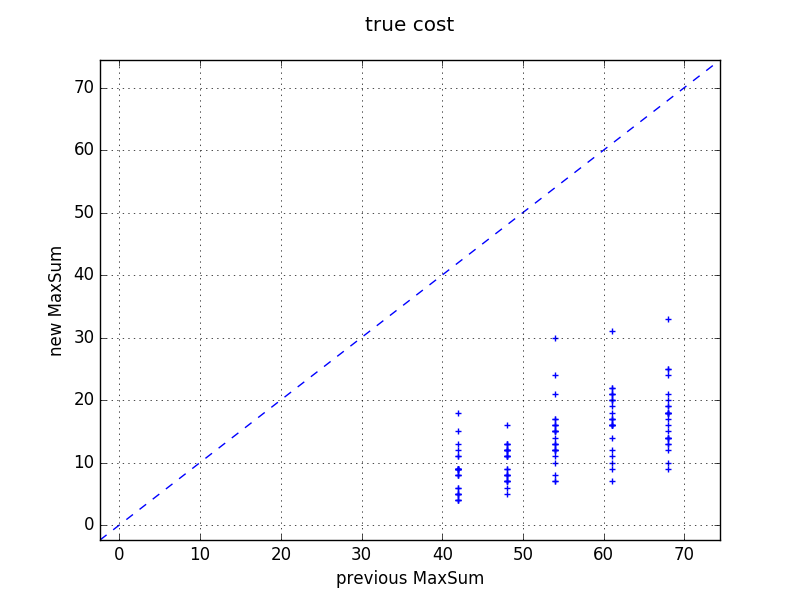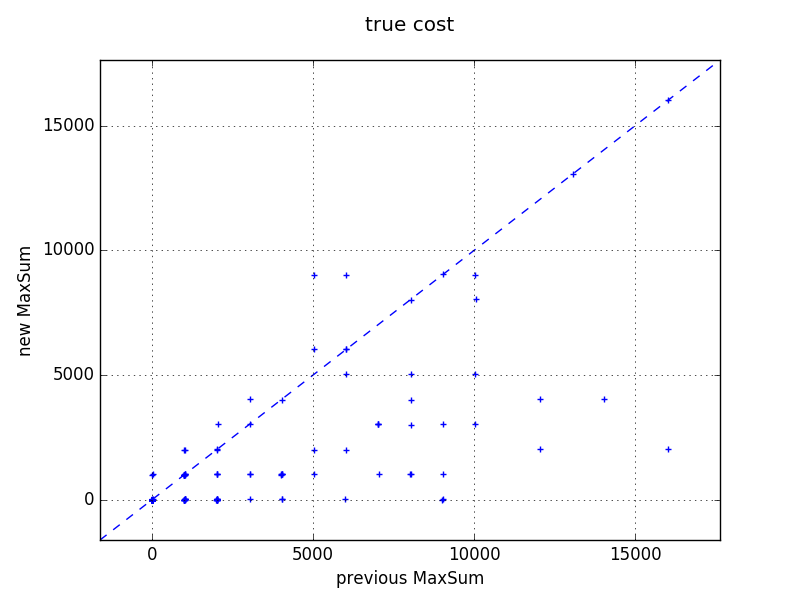## Major changes

• Max-Sum now optionally introduces perturbations to the problem to improve solution quality.
The resulting empirical improvements are reported below on graph coloring and on meeting scheduling problems.
• The distributed submode now support a non-omniscient controller, in which case the configuration files are fed directly to the daemons (support request #10 by Andrea).
• The XCSPparser's main function can now split an overall problem into each agent's respective subproblem (support request #10 by Andrea).

## Empirical performance improvements on graph coloring problems

The figure below reports the performance of Max-Sum in FRODO version 2.15 ("new MaxSum") against FRODO version 2.14 ("previous MaxSum") in solution cost on graph coloring problems, with soft constraints (violating a constraint incurs a cost of 1), varying number of nodes from 15 to 19, a density of 0.4, a tightness of 0.0 (i.e. no unary constraints), and 3 colors.In this problem domain, Max-Sum typically performs very poorly, because of the multiplicity of optimal solutions: for each node in the graph, and for each possible color for that node, there exists an optimal solution in which the node takes that color. As a result, to each variable node in the factor graph, each value (i.e. each color) looks equivalently good. In FRODO version 2.14, Max-Sum would deal with this by just arbitrarily picking the first color. As a result, all nodes end up taking the same color, and all constraints get violated, which is why Max-Sum's solution costs correspond to the number of edges in the graph.

In FRODO version 2.15, Max-Sum optionally introduces random perturbations to the problem in order to break symmetry and artificially impose the existence of a single optimal solution. These perturbations are introduced in the form of unary constraints that indicate each node's color preferences, as described in the paper below. In the experiment that produced the above results, the amplitude of the perturbations was set to 0.01 in order to remain negligible compared to the cost of a constraint violation.

Alessandro Farinelli, Alex Rogers, Adrian Petcu, and Nicholas R. Jennings. Decentralised coordination of low-power embedded devices using the max-sum algorithm. In Lin Padgham, David C. Parkes, Jörg P. Müller, and Simon Parsons, editors, Proceedings of the Seventh International Conference on Autonomous Agents and Multiagent Systems (AAMAS’08), pages 639–646, Estoril, Portugal, May 12–16 2008.

## Empirical performance improvements on meeting scheduling problems

Even on a problem domain in which there does not necessarily exist multiple optimal solutions, introducing perturbations also significantly improves the performance of Max-Sum, as illustrated in the figure below. These results were obtained on random meeting scheduling problems expressed as DCOPs using the Events As Variables (EAV) approach described in the following paper.

Rajiv T. Maheswaran, Milind Tambe, Emma Bowring, Jonathan P. Pearce, and Pradeep Varakantham. Taking DCOP to the real world: Efficient complete solutions for distributed multi-event scheduling. In Nicholas R. Jennings, Carles Sierra, Liz Sonenberg, and Milind Tambe, editors, Proceedings of the Third International Joint Conference on Autonomous Agents and Multiagent Systems (AAMAS’04), volume 1, pages 310–317, Columbia University, New York City, U.S.A., July 19–23 2004. ACM Special Interest Group on Artificial Intelligence (SIGART), IEEE Computer Society.

The problems had soft constraints with constraint violation penalties of 1000, and unary constraints expressing each agent's cost (random in [0, 10]) of having any meeting in each of the 8 time slots. The number of meetings was varied between 1 and 6, each meeting involving 2 agents randomly picked from a common pool of 3 agents. Max-Sum's perturbation amplitude was set to 0.01.